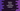# Python string isdecimal method explanation with example## Python string isdecimal() method explanation with examples:

The isdecimal() method is used to check if all characters of a string are decimal characters or not. It returns True if all characters of the string are decimal, else it returns False. All characters which can be used to form numbers in base 10 are called decimal characters. The Unicode General category of a decimal character is ‘Nd’.

Let’s learn how to use isdecimal() in python with examples.

### Definition of isdecimal:

The isdecimal method is defined as like below:

``str.isdecimal()``

### Return value of isdecimal():

isdecimal() returns one boolean value. It returns True if all the characters of the string str are decimal characters. Else it returns False.

### Example of isdecimal():

The below example shows how isdecimal() works:

``````str_list = ['helloworld', '1234', '012',
'12.34', '12 34', '-12', '+12', '123@']

for s in str_list:
print(f'{s} => {s.isdecimal()}')``````

str_list is a list of strings. It uses a for loop to iterate over the strings of the list and prints the value of isdecimal() on each string.

It will print:

``````helloworld => False
1234 => True
012 => True
12.34 => False
12 34 => False
-12 => False
+12 => False
123@ => False``````

It returns True only for the second and third strings as both includes only decimal characters.

### Find total number of decimal characters in a string:

We can use the isdecimal() method to find the total number of decimal characters in a string. We can iterate over the characters of the string one by one and use isdecimal() method with each character to find the total number of decimal characters and non-decimal characters.

Let’s take a look at the below program:

``````input_str = input('Enter a string: ')

total_decimal = 0
total_non_decimal = 0

for c in input_str:
if c.isdecimal():
total_decimal += 1
else:
total_non_decimal += 1

print(
f'Total decimal characters: {total_decimal}, Total non-decimal characters: {total_non_decimal}')``````

In this example, input_str is the variable to hold the user input string. Here, total_decimal is the variable to hold the total number of decimal characters and total_non_decimal is the variable to hold the total number of non-decimal characters.

The for loop iterate through the characters of the string one by one. For each character, it uses isdecimal() method to check if it is a decimal character or not. Based on the return value, it increments the value of total_decimal or total_non_decimal by 1.

At the end of the program, it prints the calculated count, i.e. total_decimal and total_non_decimal.

``````Enter a string: hello123
Total decimal characters: 3, Total non-decimal characters: 5

Enter a string: hello 123 world !!
Total decimal characters: 3, Total non-decimal characters: 15``````

### Example of isdecimal() with unicode:

isdecimal() can be used with unicode values. It returns True for all valid decimal string.

``````input_str = ['\u0035', '\u0036', '\u0037', '٦', '੭']

for s in input_str:
print(s.isdecimal())``````

It will print True for each of these 5 strings:

``````True
True
True
True
True``````
• \u0035 is the unicode value of digit five.
• \u0036 is the unicode value of digit six.
• \u0037 is the unicode value of digit seven.
• ٦ is digit six in Arabic
• ੭ is digit seven in Gurmukhi